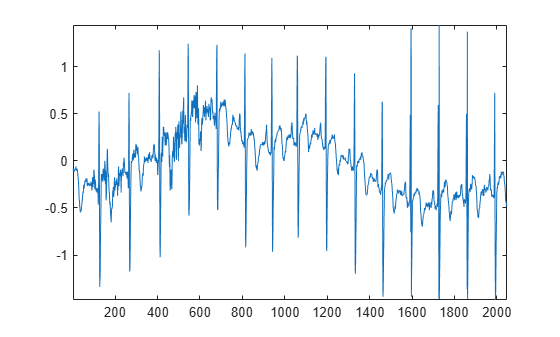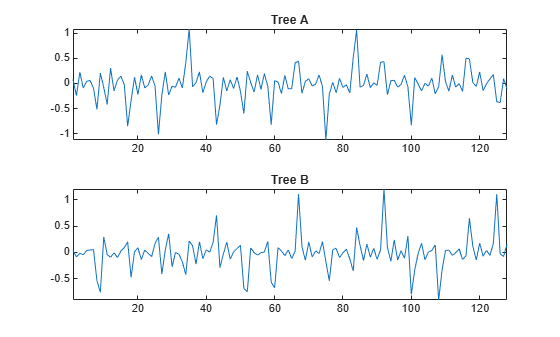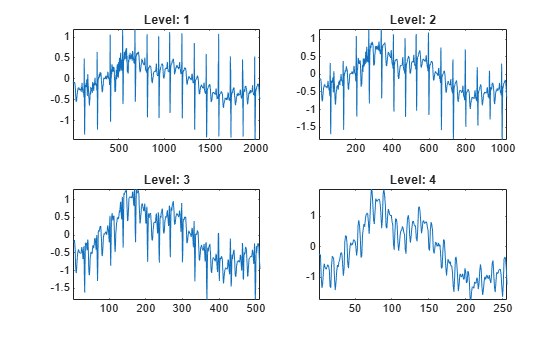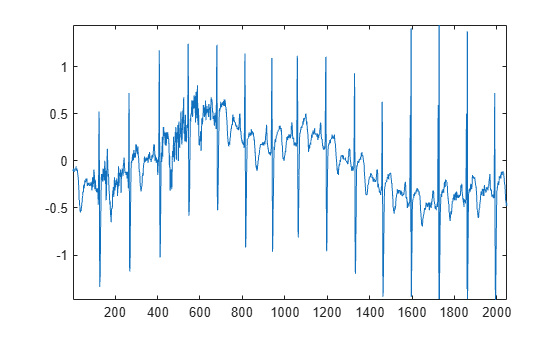# dualtree

Kingsbury Q-shift 1-D dual-tree complex wavelet transform

## Syntax

``[A,D] = dualtree(X)``
``[___,Ascale] = dualtree(X)``
``[___] = dualtree(X,Name,Value)``

## Description

````[A,D] = dualtree(X)` returns the 1-D dual-tree complex wavelet transform (DTCWT) of `X`. The output `A` is the matrix of real-valued final-level scaling (lowpass) coefficients. The output `D` is an L-by-1 cell array of complex-valued wavelet coefficients, where L is the level of the transform.The input `X` must have at least two samples. The DTCWT is obtained by default down to level `floor(log2N)`, where N is the length of `X` if `X` is a vector and the row dimension of `X` if `X` is a matrix. If N is odd, `X` is extended by one sample by reflecting the last element of `X`.By default, `dualtree` uses the near-symmetric biorthogonal filter pair with lengths 5 (scaling filter) and 7 (wavelet filter) for level 1 and the orthogonal Q-shift Hilbert wavelet filter pair of length 10 for levels greater than or equal to 2.```
````[___,Ascale] = dualtree(X)` returns the scaling (lowpass) coefficients at each level.```

example

````[___] = dualtree(X,Name,Value)` specifies additional options using name-value pair arguments. For example, `'Level',10` specifies a decomposition down to level 10.```

## Examples

collapse all

```load wecg plot(wecg) axis tight```Obtain the 4-level dual-tree transform. Return the approximation (lowpass) coefficients at all levels.

`[a,d,as] = dualtree(wecg,'Level',4);`

Plot the final-level wavelet coefficients from tree A and tree B.

```figure subplot(2,1,1) plot(real(d{4})) axis tight title('Tree A') subplot(2,1,2) plot(imag(d{4})) axis tight title('Tree B')```Plot the lowpass coefficients at each level of the transform.

```figure for k=1:4 subplot(2,2,k) plot(as{k}) axis tight title(['Level: ',num2str(k)]) end```This example shows that small signal shifts do not significantly change the distribution of energy among the DTCWT coefficients at different scales.

Load an ECG signal. The signal has 2048 samples.

```load wecg len = numel(wecg); plot(wecg) axis tight```Create two 1-by-3000 zero vectors. Insert the ECG signal into different segments of each zero vector.

```shift1 = 328; shift2 = 368; vec1 = zeros(1,3000); vec2 = zeros(1,3000); vec1(shift1+[1:len]) = wecg; vec2(shift2+[1:len]) = wecg;```

Obtain the dual-tree transform of both vectors. Use default settings.

```[a1,d1] = dualtree(vec1); [a2,d2] = dualtree(vec2);```

Compute the energy at each scale for both decompositions. Note that the energy distribution of the shifted signals across all scales remains approximately the same.

```energy1 = cell2mat(cellfun(@(x)(sum(abs(x).^2)),d1,'uni',0)); energy2 = cell2mat(cellfun(@(x)(sum(abs(x).^2)),d2,'uni',0)); levels =cell(numel(energy1),1); for k=1:numel(energy1) levels{k} = sprintf('Level %d',k); end energies = table(levels,energy1,energy2)```
```energies=11×3 table levels energy1 energy2 ____________ _______ _______ {'Level 1' } 16.014 16.014 {'Level 2' } 19.095 19.095 {'Level 3' } 35.99 35.99 {'Level 4' } 25.141 25.065 {'Level 5' } 16.81 17.452 {'Level 6' } 9.7078 9.161 {'Level 7' } 2.3201 2.0513 {'Level 8' } 8.3808 8.4197 {'Level 9' } 23.006 22.56 {'Level 10'} 70.764 73.964 {'Level 11'} 64.097 59.022 ```

## Input Arguments

collapse all

Input data, specified as a real-valued vector, matrix, or timetable. The input `X` must have at least two samples. If `X` is a timetable, it can contain a single vector or matrix variable, or it can contain multiple variables, each containing a column vector. If `X` is a matrix, `dualtree` operates on the columns of `X`.

Data Types: `double` | `single`

### Name-Value Pair Arguments

Specify optional comma-separated pairs of `Name,Value` arguments. `Name` is the argument name and `Value` is the corresponding value. `Name` must appear inside quotes. You can specify several name and value pair arguments in any order as `Name1,Value1,...,NameN,ValueN`.

Example: `'LevelOneFilter','antonini','Level',4`

Level of decomposition, specified as a positive integer less than or equal to `floor(log2N)`, where N is the length of `X` if `X` is a vector and the row dimension of `X` if `X` is a matrix. If unspecified, `Level` defaults to `floor(log2N)`.

Biorthogonal filter to use in the first-level analysis, specified as:

• `'legall'` — LeGall 5/3 filter 

• `'nearsym13_19'` — (13,19)-tap near-orthogonal filter 

• `'nearsym5_7'` — (5,7)-tap near-orthogonal filter 

• `'antonini'` — (9,7)-tap Antonini filter 

By default, `dualtree` uses `'nearsym5_7'`, the near-symmetric biorthogonal filter pair with lengths 5 (scaling filter) and 7 (wavelet filter).

Orthogonal Hilbert Q-shift analysis filter pair length to use for levels 2 and higher, specified as one of the listed values . By default, `dualtree` uses the orthogonal Q-shift Hilbert wavelet filter pair of length 10.

## Output Arguments

collapse all

Final-level approximation coefficients, returned as a real-valued vector if `X` is a vector, or a matrix if `X` is a multisignal. The approximation coefficients are the final-level scaling (lowpass) coefficients. If `X` is a matrix, the column dimensions of `X` and `A` are equal.

Wavelet coefficients, returned as an L-by-1 cell array of complex-valued wavelet coefficients, where L is the level of the transform. The real parts of the coefficients are from tree A, and the imaginary parts are from tree B. If `X` is a matrix, each element of `D` is a matrix whose column dimension equals the column dimension of `X`.

Approximation coefficients at each level of the transform, returned as an L-by-1 cell array of real-valued scaling (lowpass) coefficients, where L is the level of the transform. If `X` is a matrix, each element of `D` is a matrix whose column dimension equals the column dimension of `X`.

 Antonini, M., M. Barlaud, P. Mathieu, and I. Daubechies. “Image Coding Using Wavelet Transform.” IEEE Transactions on Image Processing 1, no. 2 (April 1992): 205–20. https://doi.org/10.1109/83.136597.

 Kingsbury, Nick. “Complex Wavelets for Shift Invariant Analysis and Filtering of Signals.” Applied and Computational Harmonic Analysis 10, no. 3 (May 2001): 234–53. https://doi.org/10.1006/acha.2000.0343.

 Le Gall, D., and A. Tabatabai. “Sub-Band Coding of Digital Images Using Symmetric Short Kernel Filters and Arithmetic Coding Techniques.” In ICASSP-88., International Conference on Acoustics, Speech, and Signal Processing, 761–64. New York, NY, USA: IEEE, 1988. https://doi.org/10.1109/ICASSP.1988.196696.

## Support